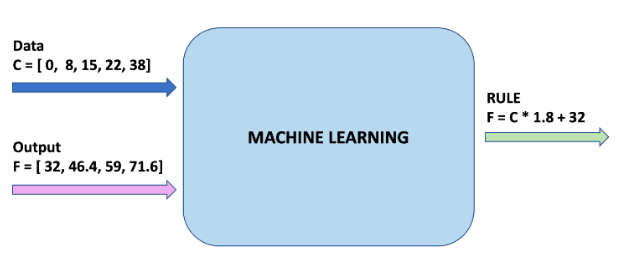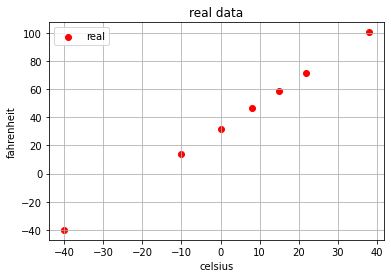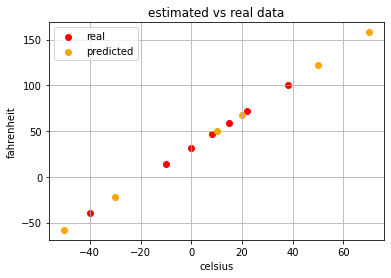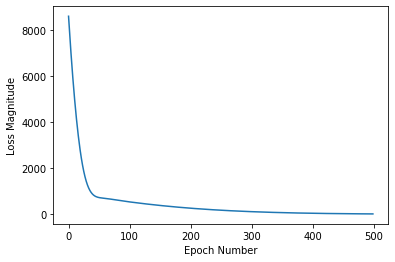# 机器学习(一)：5分钟理解机器学习并上手实践\$\$ Fahrenheit = Celsius * 1.8 + 32 \$\$import numpy as np
import matplotlib.pyplot as plt
celsius = [[-40], [-10], [ 0], [ 8], , , [ 38]]
fahrenheit = [[-40], [ 14], , [46.4], , [71.6], [100.4]]
plt.scatter(celsius,fahrenheit, c="red", label="real")
plt.xlabel("celsius")
plt.ylabel("fahrenheit")
plt.legend()
plt.grid(True)
plt.title("real data")
plt.show()from sklearn.linear_model import LinearRegression
lr = LinearRegression()
lr.fit(celsius,fahrenheit)

celsius_test = [[-50],[-30],,,,]
fahrenheit_test = lr.predict(celsius_test)
plt.scatter(celsius,fahrenheit, c="red", label="real")
plt.scatter(celsius_test,fahrenheit_test, c="orange", label="predicted")
plt.xlabel("celsius")
plt.ylabel("fahrenheit")
plt.legend()
plt.grid(True)
plt.title("estimated vs real data")
plt.show()import tensorflow as tf
import numpy as np
# prepare data
celsius_q = np.array([-40, -10, 0, 8, 15, 22, 38], dtype=float)
fahrenheit_a = np.array([-40, 14, 32, 46.4, 59, 71.6, 100.4], dtype=float)
# fit model
model = tf.keras.Sequential([tf.keras.layers.Dense(units=1, input_shape=)])
model.compile(loss="mean_squared_error", optimizer=tf.keras.optimizers.Adam(0.1))
history = model.fit(celsius_q, fahrenheit_a, epochs=500, verbose=False)
print("Finished training the model")
# print loss
import matplotlib.pyplot as plt
plt.xlabel("Epoch Number")
plt.ylabel("Loss Magnitude")
plt.plot(history.history["loss"])## ZooKeeper实现生产-消费者队列

【欢迎关注公众号：程序猿讲故事（codestory），及时接收最新文章】生产-消费者队列，用于多节点的分布式数据结构，生产和消费数据。生产者创建一个数据对象，并放到队列中；消费者从队列中取出一个数据对...

## index match函数的使用方法_INDEX与MATCH函数嵌套使用

WPS表格中经常会使用到函数，其中INDEX函数是很常用的查找引用函数，通常情况下会嵌套其他函数一起使用，比如“index+match”今天我们就通过...

## altium designer安装步骤_电动汽车高压电池拆装没有那么难，一文教你详细步骤

Part1  需要的工具及注意事项  以比亚迪新能源车型为例，高压电池模组拆卸所需设备及工具如下图所示。高压电池属于高压危险产品，维修人员拆装过程需注意以下事项࿱...